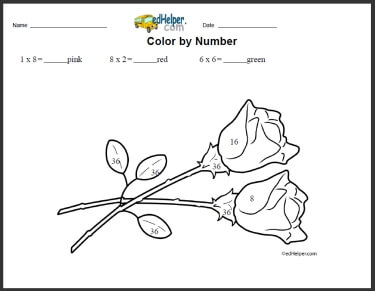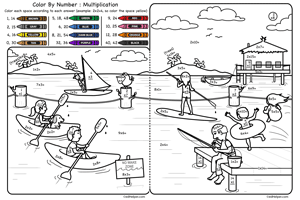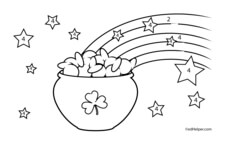Worksheets and No Prep Teaching Resources
Math Worksheets

# Free Color by Multiplication Worksheets

Multiplication facts will be a breeze when combined with fun coloring sheets that will fascinate students. These creative worksheets will help students hone the important skill of multiplication that is necessary for future math mastery. Students will love finishing a colorful piece of art while effortlessly polishing their multiplication skills.Color by Number MultiplicationColor by Number: Multiplication

Math Lesson: Color by Numbers - February Color by Number BookMultiplication Coloring: a multiplication equation given for each color Multiplication and Division Coloring: a multiplication or division equation given for each color

Math Lesson: Color by Numbers - March Color by Number BookMultiplication Coloring: a multiplication equation given for each color Multiplication and Division Coloring: a multiplication or division equation given for each color

Math Lesson: Color by Numbers - August Color by Number BookMultiplication Coloring: a multiplication equation given for each color Multiplication and Division Coloring: a multiplication or division equation given for each color

Have a suggestion or would like to leave feedback?